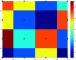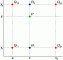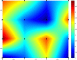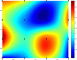I have forgotten

•http://facebook.com/
•https://www.google.com/accounts/o8/id
•https://me.yahoo.com

# Interpolation

#### Akima

Interpolates a given set of points using Akima spline fitting.
 double Akima_once (int N, double *x, double *y, double a)#### Cubic

Interpolates a given set of points using cubic spline fitting.
 double Cubic_once (int N, double *x, double *y, double a)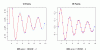#### Lagrange

Interpolates a given set of points using the Lagrange polynomial.
 double Lagrange_once (int N, double *x, double *y, double a, int l)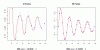#### Linear

Linearly interpolates a given set of points.
 double Linear_once (int N, double *x, double *y, double a)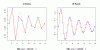#### Univariate

Univeriate curve-fitting, interpolation, polynomial, spline, Akima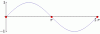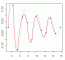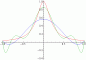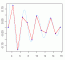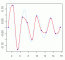#### Multivariate

Multivariate interpolation, nearest-neighbor, bilinear, multilinear, bicubic, multicubic